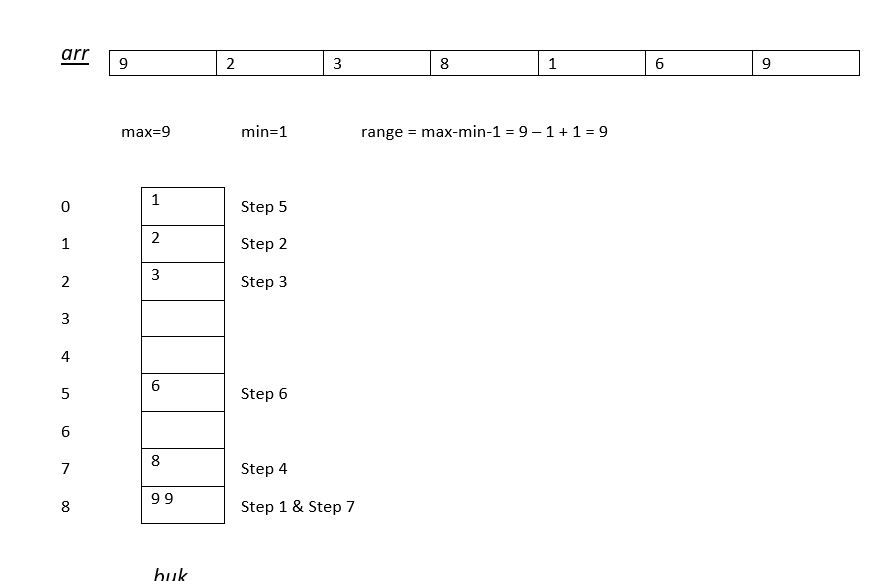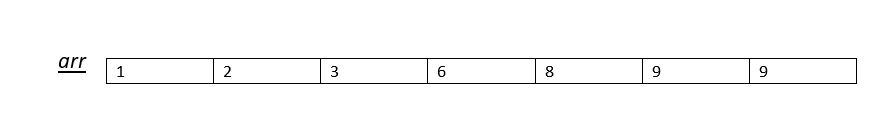×

Search anything:

# Pigeonhole Sort

#### Algorithms Sorting Algorithms pigeonhole sortingGet this book -> Problems on Array: For Interviews and Competitive Programming

Reading time: 20 minutes | Coding time: 5 minutes

Pigeonhole sorting is a sorting algorithm that is suitable for sorting lists of elements where the number of elements and the number of possible key values are approximately the same.
Where key here refers to the part of a group of data by which it is sorted, indexed, cross referenced, etc.It is used in quick, heap and many other sorting algorithm.
If we consider number of elements (n) and the length of the range of possible key values (N) are approximately the same. It requires O(n + N) time.

### Working of Pigeonhole Sort

1. Find minimum and maximum values in array. Let the minimum and maximum values be ‘min’ and ‘max’ respectively. Also find range as max-min+1.

2. Set up an array of initially empty “pigeonholes” the same size as of the range calculated above.

3. Visit each element of the array and then put each element in its pigeonhole. An element arr[i] is put in hole at index arr[i] – min.

4. Start the loop all over the pigeonhole array in order and put the elements from non- empty holes back into the original array.

In Pigeonhole sort, we need not compare two data to sort them and hence, it is an in-place sorting algorithm

### ExampleAs you can see a lot of blocks are empty, this algorithm requires a lot of space even for a small but widely spread data.

Explaining the steps mentioned above :

When we implement point 3 in working of Pigeonhole Sort we do the following :

Step 1 : 9-1 = 8 => buk = arr
Step 2 : 2-1 = 1 => buk = arr
Step 3 : 3-1 = 2 => buk = arr
Step 4 : 8-1 = 7 => buk = arr
Step 5 : 1-1 = 0 => buk = arr
Step 6 : 6-1 = 5 => buk = arr
Step 7 : 9-1 = 8 => buk = arr

After implementing the array we get :### Difference between Counting and Pigeonhole sort

The ONLY difference between counting and pigeonhole sort is that in the secondary sort we move items twice i.e one on the input array and other in the bucket array. While in first sort we only move the items once.

• C++
• Python

### C++

``````
#include <bits/stdc++.h>
using namespace std;
/* Sorts the array using pigeonhole algorithm */
void pigeonholeSort(int arr[], int n)
{
// Find minimum and maximum values in arr[]
int min = arr, max = arr;
for (int i = 1; i < n; i++)
{
if (arr[i] < min)
min = arr[i];
if (arr[i] > max)
max = arr[i];
}
int range = max - min + 1; // Find range
// Create an array of vectors. Size of array
// range. Each vector represents a hole that
// is going to contain matching elements.
vector holes[range];
// Traverse through input array and put every
// element in its respective hole
for (int i = 0; i < n; i++)
holes[arr[i]-min].push_back(arr[i]);
// Traverse through all holes one by one. For
// every hole, take its elements and put in
// array.
int index = 0;  // index in sorted array
for (int i = 0; i < range; i++)
{
vector::iterator it;
for (it = holes[i].begin(); it != holes[i].end(); ++it)
arr[index++]  = *it;
}
}
// Driver program to test the above function
int main()
{
int arr[] = {9,2,3,8,1,6,9};
int n = sizeof(arr)/sizeof(arr);
pigeonholeSort(arr, n);
printf("Sorted order is : ");
for (int i = 0; i < n; i++)
printf("%d ", arr[i]);
return 0;
}``````

### Python

``````
def pigeonhole_sort(a):
# size of range of values in the list
# (ie, number of pigeonholes we need)
my_min = min(a)
my_max = max(a)
size = my_max - my_min + 1
# our list of pigeonholes
holes =  * size
# Populate the pigeonholes.
for x in a:
assert type(x) is int, "integers only please"
holes[x - my_min] += 1
# Put the elements back into the array in order.
i = 0
for count in range(size):
while holes[count] > 0:
holes[count] -= 1
a[i] = count + my_min
i += 1
a = [9,2,3,8,1,6,9]
print("Sorted order is : ", end = ' ')
pigeonhole_sort(a)
for i in range(0, len(a)):
print(a[i], end = ' ')
``````

### Complexity

• Worst case time complexity: `Θ(n+N)`
• Average case time complexity: `Θ(n+N)`
• Best case time complexity: `Θ(n+N)`
• Space complexity: `Θ(n+N)`

where n is the number of elements and N is the range of the input data

### Question

#### Which is more efficient Bucket sort or Pigeonhole sort for larger value of N?

Bucket sort
Pigeon sort
Both are equally efficient
Depends on the machine
For arrays where N is much larger than n, bucket sort is a generalization that more efficient in space and time.#### Harshita Sahai

Maintainer at OpenGenus | Previously Software Developer, Intern at OpenGenus (June to August 2019) | B.Tech in Information Technology from Guru Gobind Singh Indraprastha University (2017 to 2021)